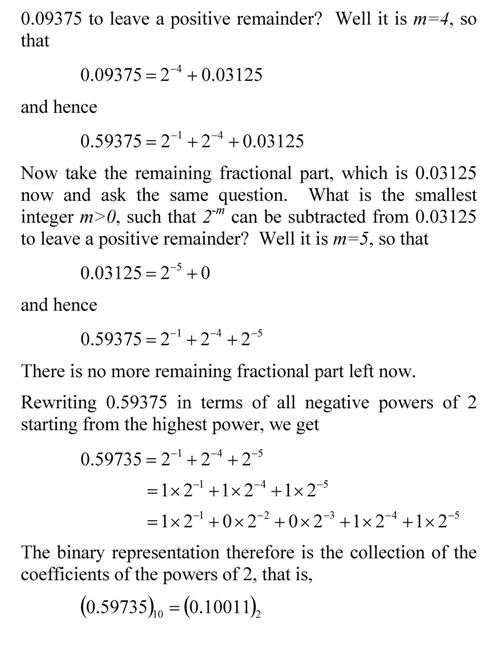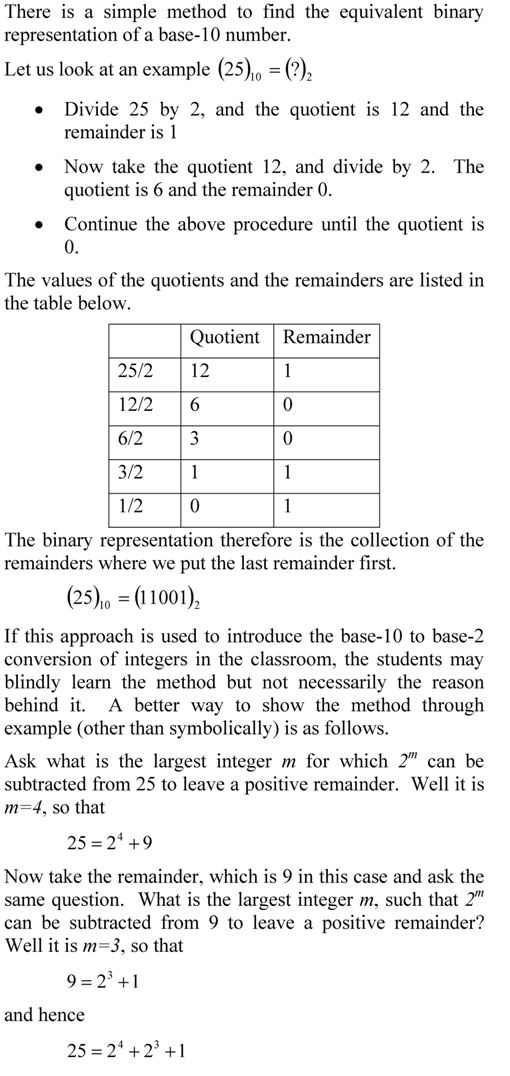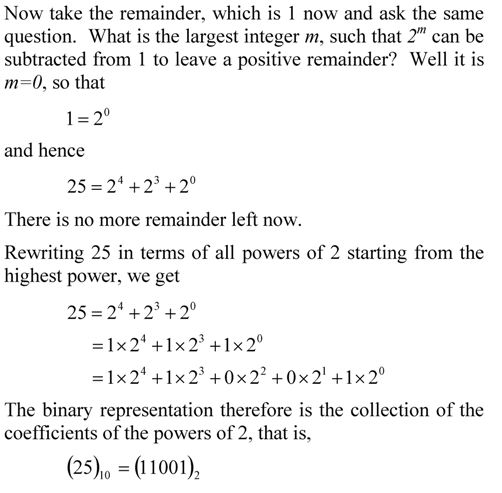## Largest integer that can be represented in a n-bit integer word

To find the largest integer in base-10 that can be represented in an n-bit integer word, let’s do this inductively.

• If you have 3 bit-word, the highest number is (111) of base-2 which is 7 (1*2^2+1*2^1+1*2^0) of base-10,
• If you have 4 bit-word, the highest number is (1111) of base-2 which is 15 (1*2^3+1*2^2+1*2^1+1*2^0) of base-10,
• if you have 5 bit-word, the highest number is (11111) of base-2 which is 31 (1*2^4+1*2^3+1*2^2+1*2^1+1*2^0) of base-10.

There is a trend here: 3 bit-word stores a maximum number of 7 (2^3-1), 4-bit word stores a maximum of 15 (2^4-1), 5 bit-word store a maximum number of 31 (2^5-1), and so on.   This means that the maximum number stored in n-bit word stores a maximum number of 2^n-1.

We can derive the maximum number by knowing that the maximum base-10 number in a n-bit word is the summation series:
1*2^(n-1)+1*2^(n-2)+………+1×2^0.
This is a geometric progression series.  The formula for the sum of a geometric series
a+ar+ar^2+…+a*r^n =a*(1-r^(n+1))/(1-r), r ≠ 1,
Hence,
1*2^(n-1)+1*2^(n-2)+………+1×2^0=1*(1-2^(n))/(1-2)=2^n-1 _____________________________________________________________

This post is brought to you by Holistic Numerical Methods: Numerical Methods for the STEM undergraduate at http://numericalmethods.eng.usf.edu, the textbook on Numerical Methods with Applications available from the lulu storefront, the textbook on Introduction to Programming Concepts Using MATLAB, and the YouTube video lectures available at http://numericalmethods.eng.usf.edu/videos.  Subscribe to the blog via a reader or email to stay updated with this blog. Let the information follow you.

## A Wolfram demo on converting a decimal number to floating point binary representation

Here is another Wolfram demo. This one converts a decimal number to a floating point binary representation.  To play with the demo, download the free CDF player first.

The total number of bits used for the representation =
one bit for the sign of the number +
one bit for the sign of the exponent +
number of bits for the exponent +
number of bits for the mantissa +
As an example, how would 54.75 be represented in a 9-bit register where the first bit is used for the sign of the number, second bit is used for sign of exponent, next three bits are used for the exponent, and the last four bits are used for the mantissa?Both the number and the exponent are positive.
As the number is normalized to lie between 1 and 2 (the interval being half-closed at the bottom and half-open at the top), the leading binary digit is always 1. So we do not actually use it in the representation of the mantissa. Hence the mantissa bits are 1011. Moreover the exponent bits are 101, the sign of the number bit is 0, and the sign of the exponent bit is 0.
Therefore the representation is.

Reference: Floating Point Representation

This post is brought to you by

Holistic Numerical Methods: Numerical Methods for the STEM undergraduate at http://numericalmethods.eng.usf.edu, the textbook on Numerical Methods with Applications available from the lulu storefront, the textbook on Introduction to Programming Concepts Using MATLAB, and the YouTube video lectures available at http://numericalmethods.eng.usf.edu/videos.  Subscribe to the blog via a reader or email to stay updated with this blog. Let the information follow you.

## Converting large numbers into floating point format by hand__________________________________________________

This post is brought to you by Holistic Numerical Methods: Numerical Methods for the STEM undergraduate at http://numericalmethods.eng.usf.edu, the textbook on Numerical Methods with Applications available from the lulu storefront, and the YouTube video lectures available at http://numericalmethods.eng.usf.edu/videos and http://www.youtube.com/numericalmethodsguy

## A better way to show conversion of decimal fractional number to binaryThis post is brought to you by Holistic Numerical Methods: Numerical Methods for the STEM undergraduate at http://numericalmethods.eng.usf.edu, the textbook on Numerical Methods with Applications available from the lulu storefront, and the YouTube video lectures available at http://www.youtube.com/numericalmethodsguy.